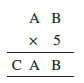# Q6.Find the values of the letters in each of the following and give reasons for the steps involved.Here , multiplication of B and 5 gives a number whose ones digit is B. this is possible when B = 0 or 5

let B = 5

B * 5 = 5 * 5 = 25

Carry = 2

5*A + 2 = CA  , This os possible only when A = 2 or 7

when A = 2 ,

5*2 + 2 = 12 which implies C = 1

when A = 7

5*7 + 2 = 37 which implies C = 3

now  B = 0

B*5 = 0*5 = 0

Carry = 0 so

5 * A = CA which is possible when A = 0 or 5 Howerver A cannot equal to 0 since AB is a two digit number

so A = 5

5*5 = 25 which implies C = 2

hence possible values of A B and C are

A = 5, 2, 7 , B = 0, 5, 5 and C = 2, 1, 3

## Related Chapters

### Preparation Products

##### JEE Main Rank Booster 2021

This course will help student to be better prepared and study in the right direction for JEE Main..

₹ 13999/- ₹ 9999/-
##### Rank Booster NEET 2021

This course will help student to be better prepared and study in the right direction for NEET..

₹ 13999/- ₹ 9999/-
##### Knockout JEE Main April 2021 (Easy Installments)

An exhaustive E-learning program for the complete preparation of JEE Main..

₹ 4999/-
##### Knockout NEET May 2021

An exhaustive E-learning program for the complete preparation of NEET..

₹ 22999/- ₹ 14999/-